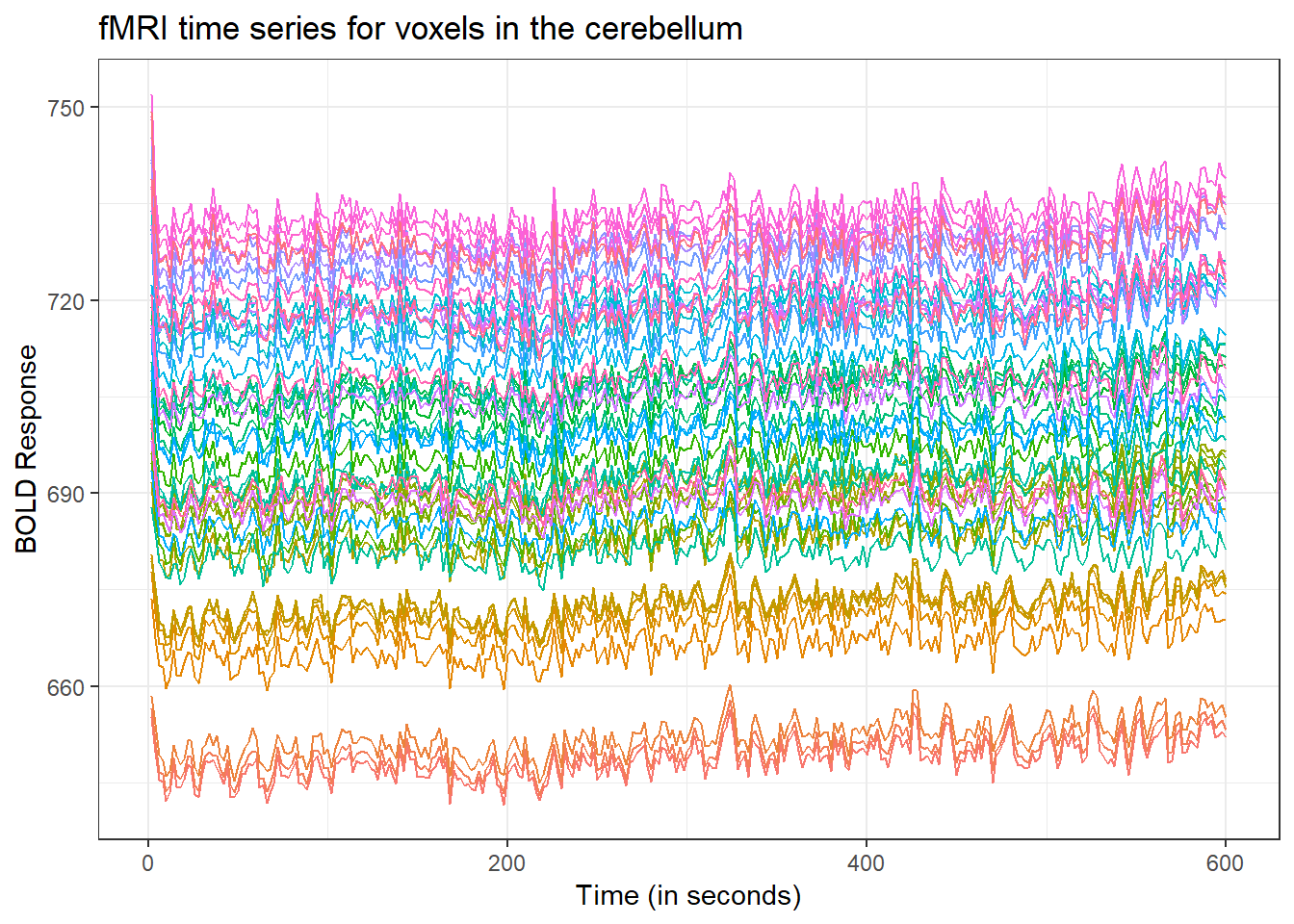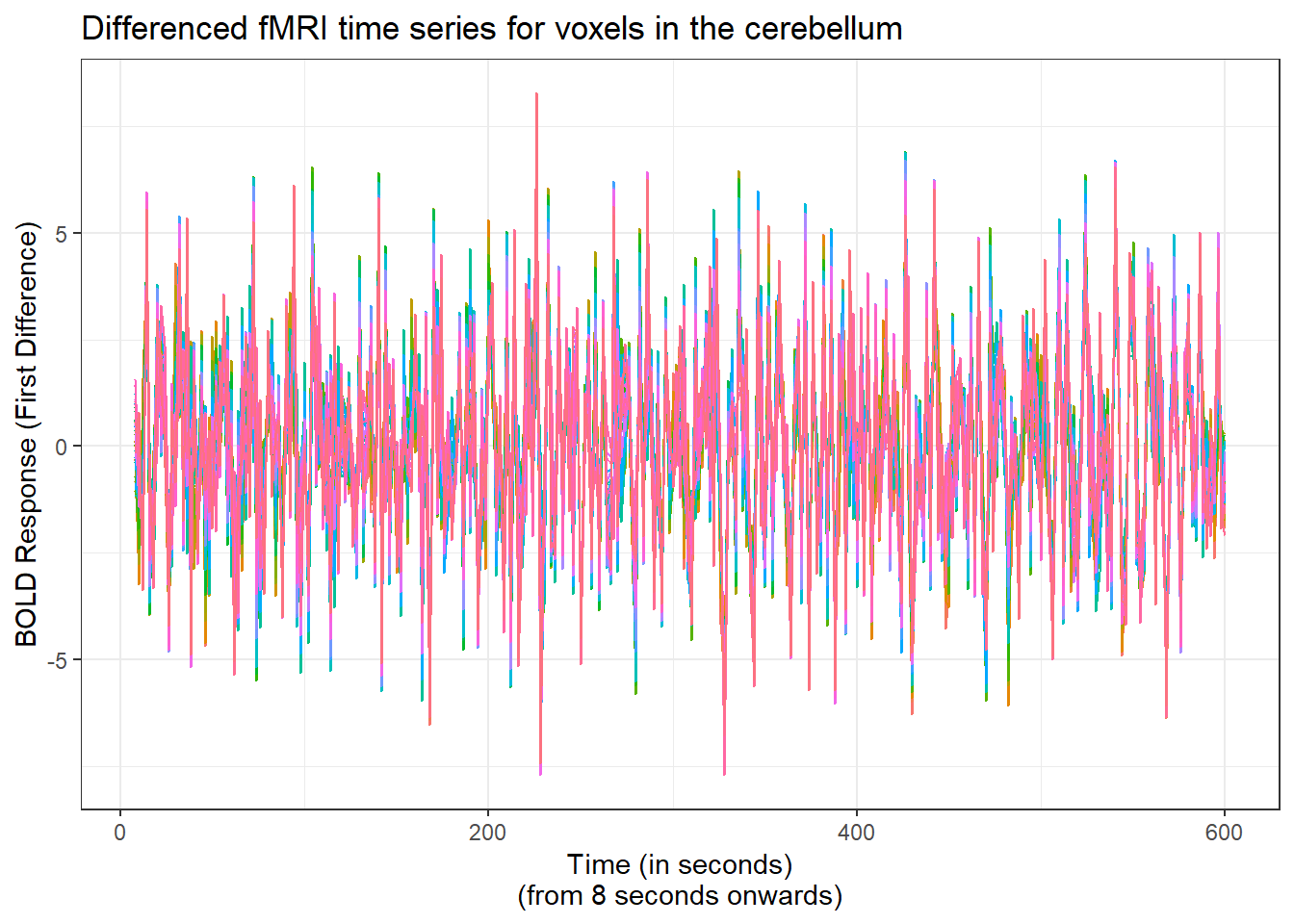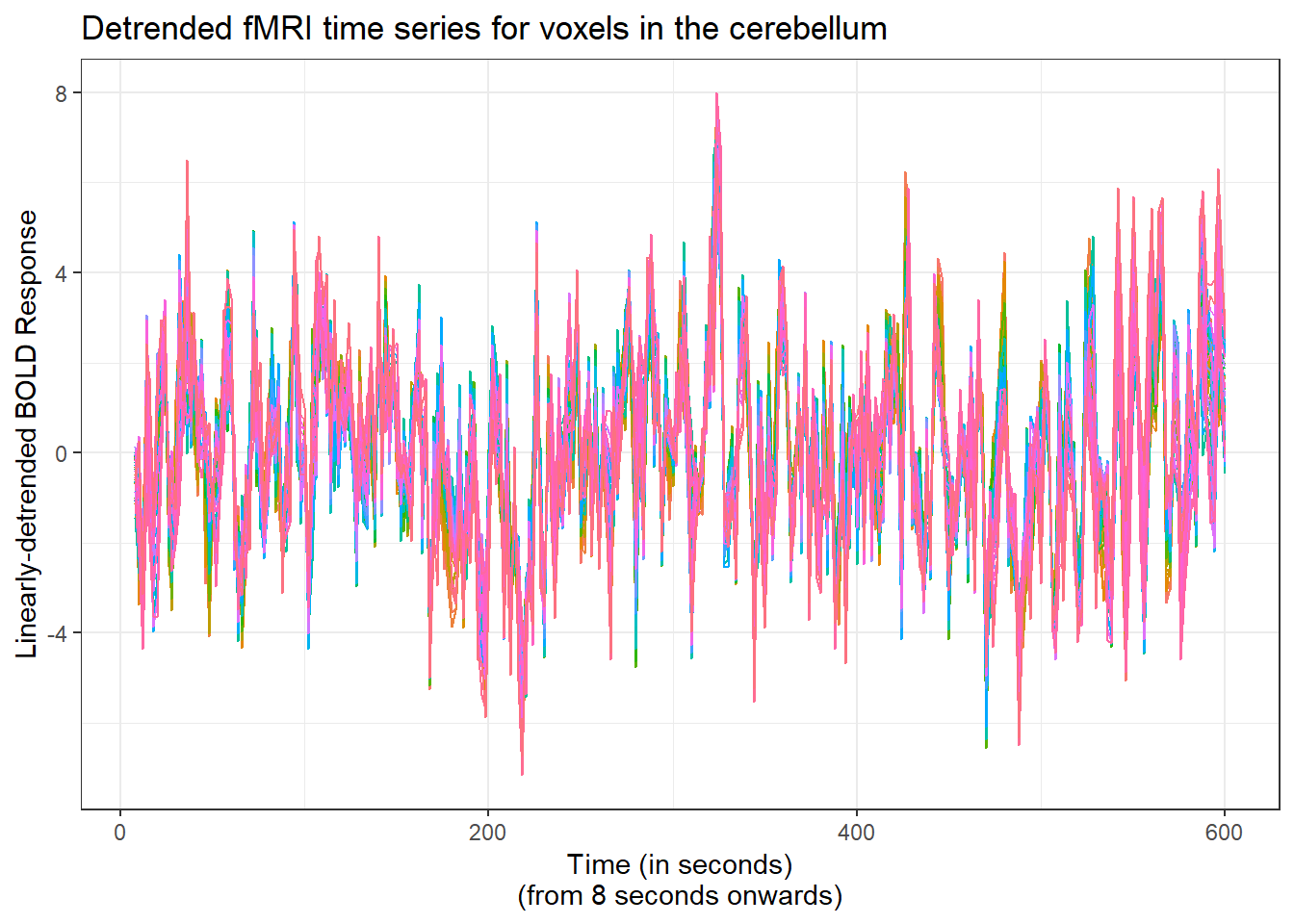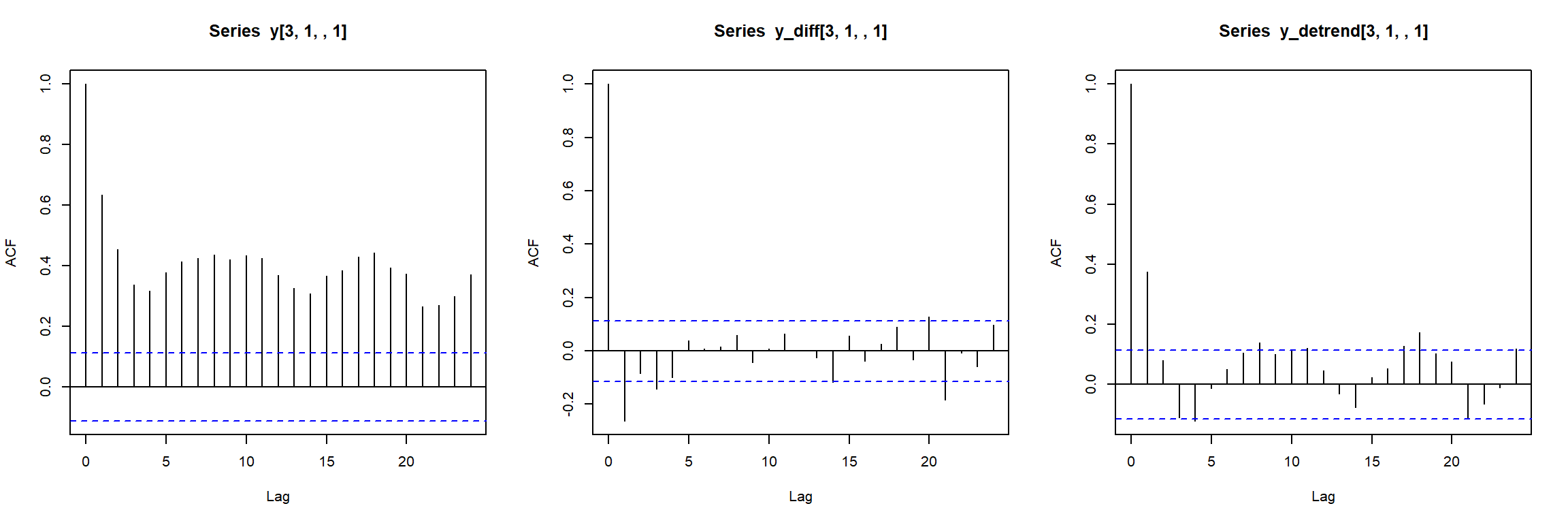Working with fMRI data, there are a large number of hurdles to overcome in order to find the signal (which voxels within an fMRI scan are associated with a thought process, a.k.a. activation) within all of the noise (observed variability) in a given scan. For example, the time series for voxels in the cerebellum for a scan from subject 1 in the Balloon Analog Risk Task Experiment (BART) data appear as follows:This shows that most of the voxels follow the same general pattern: The BOLD response is initially high, then drops down in the first 5 seconds, and then proceeds to slowly climb throughout the rest of the series. This is interesting behavior, and some people (Huettel et al, 2004) believe that it arises from scaner instability, while others believe that it may convey other meaning, at least in resting state fMRI ( Wang et al.). This trend may be a problem when trying to obtain estimate the activation in an fMRI scan.

One of the common methods used to produce a stationary time series out of a nonstationary one is to take the first difference of the data at each voxel ($$\mathbf{y}_{2:T} - \mathbf{y}_{1:(T - 1)}$$).While this create a more stationary time series, it reduces the interpretability of any inference about coefficients in a model. Rather than accounting for a change in the BOLD response, the coefficients are now interpreted as a change in the change in the BOLD response. This reduces the contrast-to-noise ratio, and therefore reduces the power of a statistical analysis.

Another idea is to detrend the data through regression. In it’s simplest form, a linear regression is used, regressing the BOLD response on it’s scan time ($$y_{t} = \beta_0 + \beta_1 t + \epsilon_t$$), and then taking the residuals from the regression as detrended data that can be used in model fitting. Here’s the linear detrend of the cerebellum data:It’s clear that the method produces different results. One way to compare them is to examine the autocorrelation function (acf) plots for the different methods. Here are the acf plots for the three different methods at one voxel location:Clearly, the differencing and detrending do much to alleviate issues of temporal dependence. Next, it may be worthwhile to see how the three data treatments perform in an activation analysis.

# Effects on activation coefficients

The data from the cerebellum was taken to be analyzed for activation with the task-based covariate. Each voxel in the frontal lobe was regressed individually, so that there is no spatial correlation inherent in such a model. Any spatial patterns are found independently within the data. The activation patterns for the frontal lobe under the three data treatments appear below: